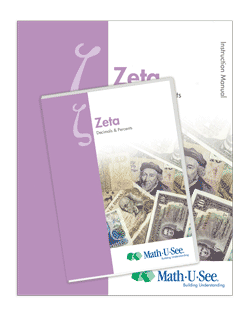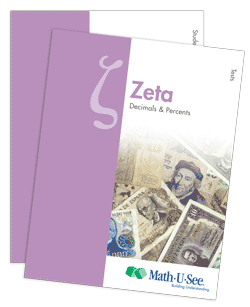# Zeta## Updated Zeta — Available March 2013

Decimals, Percents, and Other Topics

Zeta extends the student’s concept of place value to the right of the decimal point. Students learn to complete core operations with decimals. The connection between fractions and decimals is presented.Updates to Instruction Manual include introduction to:

• idea of negative numbers (no operations with negative numbers)
• idea of absolute value
• statisticsNew Application & Enrichment pages in the Student Workbook
include a focus on four main topics:

• the language of mathematics
• defining and solving ratios with charts and tables
• negative numbers on number lines and coordinate graphs
• statistics

Major Concepts and Skills Include:

• Expanding understanding of place value from positive powers of ten to include decimals
• Fluently adding, subtracting, multiplying, and dividing multiple digit decimals using place-value strategies
• Solving real-world problems with decimals and percentages
• Understanding the metric system and converting from one unit of measure to another

• Understanding and simplifying exponents
• Understanding negative numbers and representing them on the coordinate plane
• Using properties of operations to simplify and evaluate algebraic expressions
• Interpreting and graphing relationships between dependent and independent variables
• Understanding of plane geometry & geometric symbols
• Using ratio reasoning to solve problems

Please check back in the first week of March for ordering information.

Live Chat

9 AM to 6 PM Eastern time,
Monday through Friday

Phone Support

Call (888) 854-6284
International callers —
call +1 717 283 1448

8:30 AM to 6 PM Eastern time,
Monday through Friday

Email